# Similarity

(diff) ← Older revision | Latest revision (diff) | Newer revision → (diff)

A transformation of a Euclidean space in which for any two pointsandand their transforms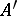and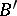one has, whereis a positive number called the similarity coefficient.

Any homothety is a similarity. An isometric mapping (including the identity) can also be regarded as a similarity with coefficientequal to one. A figureis said to be similar to a figureif there exists a similarity under which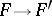. Similarity of figures is an equivalence relation, i.e. it has the properties of reflexivity, symmetry and transitivity. A similarity is a one-to-one mapping of a Euclidean space onto itself; a similarity also retains the order of points on a straight line, i.e. if a pointlies between pointsand, while,and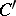are the corresponding images under some similarity, thenlies betweenand; points not lying on a straight line, under any similarity, become points not lying on one straight line. A similarity transforms a straight line into a straight line, a segment into a segment, a ray into a ray, an angle into an angle, and a circle into a circle. Under a similarity, an angle retains its value.

A similarity with coefficient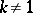that transforms each straight line into a straight line parallel to it is a homothety with coefficientor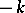. Any similarity can be considered as a composition of a motionand some homothetywith a positive coefficient.

A similarity is called proper (improper) if the motionis proper (improper). A proper similarity retains the orientation of a figure, while an improper similarity reverses the orientation.

Analogously, one defines a similarity (with retention of the above properties) in the-dimensional Euclidean space, and also in the-dimensional Euclidean and pseudo-Euclidean spaces.

A similarity is defined in an-dimensional Riemannian, pseudo-Riemannian or Finsler space as a transformation that converts the metric of the space into itself up to a constant factor.

The set of all similarities of the-dimensional Euclidean, pseudo-Euclidean, Riemannian, pseudo-Riemannian, or Finsler space constitutes an-dimensional Lie transformation group, which is called the group of similarity (homothetic) transformations for the corresponding space. In each of the spaces of these types, the Lie group of similarity transformations withparameters contains a normal subgroup of isometries of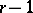dimensions.

Interest arises in metric spaces of vector densities with groups of similarities and isometries containing infinite-dimensional subgroups of isometries with common trajectories.